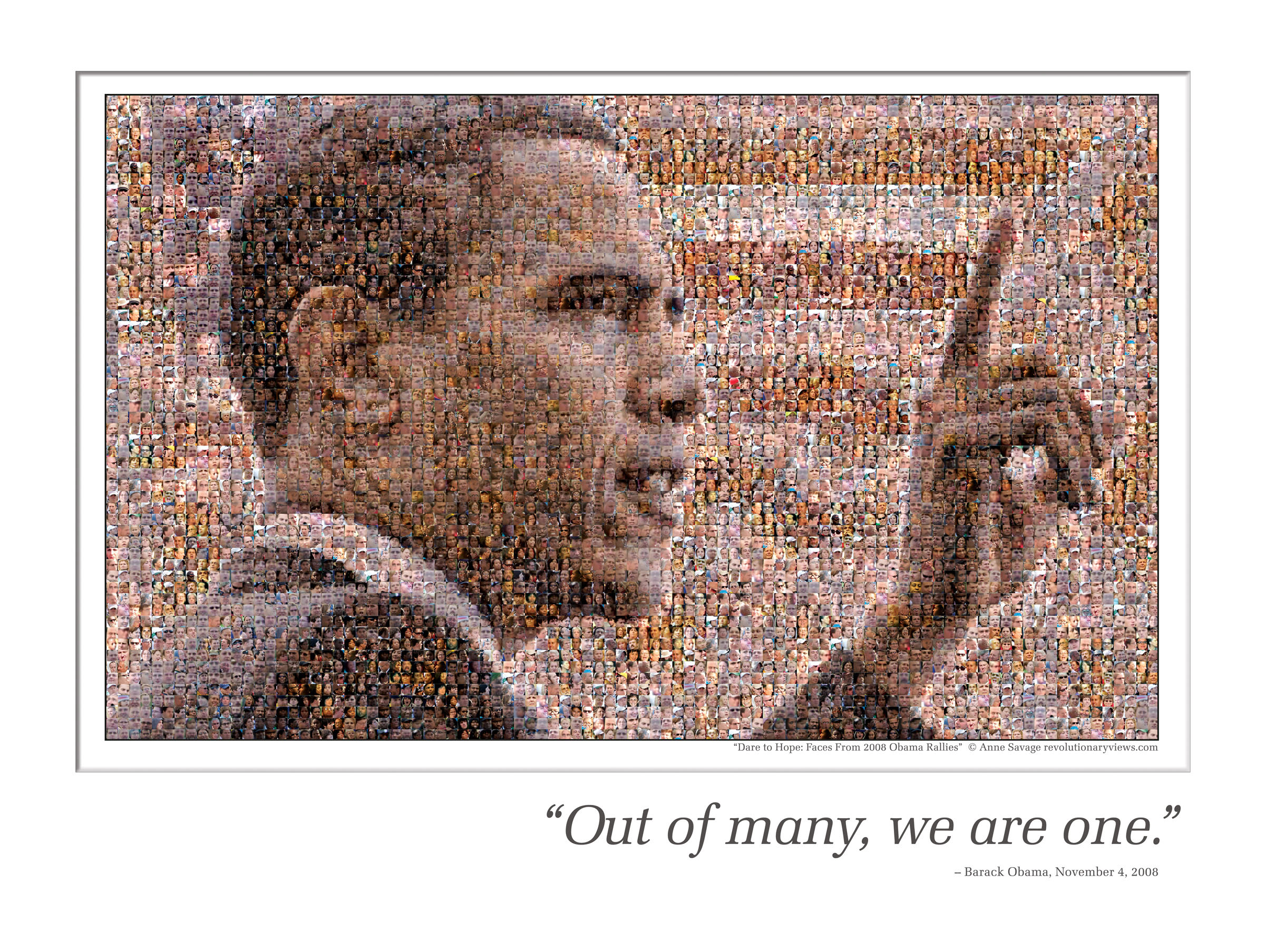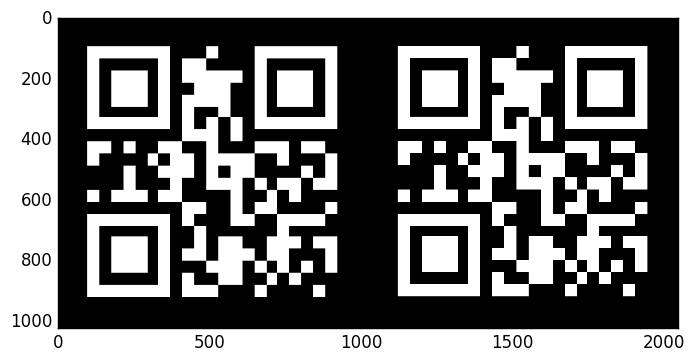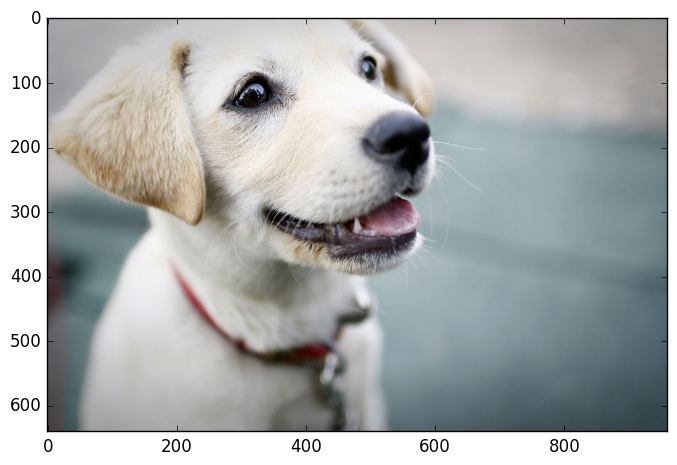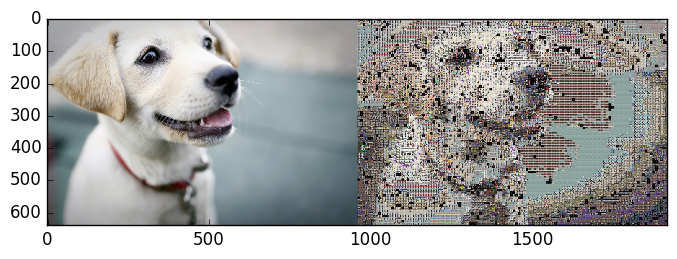# Image Mosaicking¶

## 1. Introduction¶

Mosaicking is an old art technique where pictures or designs are formed by inlaying small bits of colored stone, glass, or tile. These small bits are visible close up, but the boundaries of the bits will blend and a single recognizable image will show at a distance.

In the modern digital world, this acient art form has been transformed and combined with new technologies. As one has access to large public image database, individual images, instead of pure-colored blocks can be used as tiles to make pictures. Here is a famous mosaic image of president Obama made by Anne Savage during his campaign.Nearly 6,000 images of individual faces are used to create this image. Sure enough, it has become a very popular poster because of the special art form. Using image mosaicking to form various pictures is very cool and seems like a daunting task because we are picking and arranging thousands of pictures from a even bigger pool of pictures. There are businesses helping you create your own mosaic work with charge. However, with intuition and just a little knowledge of optimization, we can do it at home ourselves!

We know images consist of pixels, and each pixel can be described using RGB color intensities. In order for two images to look alike, we just need to match their pixels. In this case, for the image to show at a distance, intuitively, we can just treat the basis images as "super pixels" and try to match them with the corresponding subimage of the target image. Naturally, we will take the target image and partition it into a grid, where each grid element is our tile size. Then for each grid, we will choose one image from the basis that resembles the most. The way to qualify "resemblance" is discussed in detail in Part II - there are many ways of doing this. This project explores a few different ways of doing this. Because each chosen basis image resembles the grid best, after processing all grids, we will end up with a mosaicking image that overall best resembles the whole image. Notice that this is the most intuitive way to solve the problem, and it will always achieve the best result. We will present the solution using this method in the first section of Part III. This brute force method is limited by its speed; variants of the algorithm that speeds the process up will be discussed. We will also explore how changing the size of the tiles will affect the performance. The image basis in this project are obtained from online source mazaika and generic results from Google Image Search.

Disclaimer: All images are property of their respective owners. Their usage in this report is purely educational.

### What will I learn from this report?¶

• How to use Optimization techniques to create Image Mosaics
• Different techniques and the factors that affect the quality of the Image Mosaic
• How to create Image Mosaics with varying "basis" image dimensions
• Extensions to this project that you can try on your own

## 2. Mathematical model - Mixed Integer Program Formulation¶

From the introduction above, it is clear that intuitive approach to image mosaicking is an assignment problem which can be modeled as a Mixed Integer Program. Let's setup the problem as follows:

• A set of "basis" images - these are the images we will use as tiles to reconstruct the so-called "test" image (which we are trying to reporoduce). The set of "basis" images constitute the set $\mathbf{B}$
• Create a grid of equally sized sub-images from the target image - each sub-image will be a grid element. The smaller we make the grid element, the higher the quality of the resulting mosaic will be. The set of all the grid elements constitute the set $\mathbf{I}$.
• Of course, the quality also depends on the diversity of colors in our "test" image and the diversity of colors in our "basis" set.
• The size of the grid elements and the size of the "basis" images must be the same to be consistent (each grid-element is a tile location and each "basis" image is a tile that needs to fit into a tile location). Let this size be represented by $s$.

The decision variables $G_i,i\in\mathbf{I}$ ($\mathbf{I}$ is the set of all grid elements in target image) are which image (represented by its index $j\in\mathbf{B}$, where $\mathbf{B}$ is the set of basis images of size $s$) to choose for each grid $i$ of the target image. Therefore, $\mathbf{B}$ represent the restricted set of values $G_i$ can take (in other words, we can choose exactly ONE basis image as the tile to be placed in ONE grid-element). In the mathematical formulation, binary variable for each basis images is introduced to indicate whether a particular basis image is chosen for a grid-element location. For a single grid-element, the variables that indicate assignment of a basis image to that grid-element must satisfy the SOS constraint (only one image can be chosen). The objective is to minimize the visual difference$^*$ between the chosen mosaic and the target image. Note the difference function should be linear for the model to be MIP.

($^*$ Several non-linear functions can be used to describe the resemblance of the grid and the basis image and be implemented within special algorithms. But they fall out of the scope of this course and are not discussed here.)

It is worth pointing out that in the current formulation, the optimization of each grid is independent from one another. Therefore, we can lessen the solvers burden by forming a model that solves the sub-problem of optimizing each grid element. To optimize the whole target image, one can simply put the optimization model in a function and call it for each grid element of the image. Let's use the following notation:

• $m$ represents the size of a grid-element along the Y-axis (same as the height of a basis image)
• $n$ represents the size of a grid-element along the X-axis (same as the width of a basis image)

For grid-element size $m \times n$, each target grid-element can be represented by $m\times n\times 3$ RGB matrix $G$. Before optimization, basis images are pre-processed so that they all have the same size as the grid-elements. Each basis image is represented as $m\times n\times 3$ RGB matrix $B_j,j\in\mathbf{B}$. There are many different ways to quantify the similarity/dis-similarity between any basis and the target sub-image.

In this project, we have limited the grid sizes to $2^p\times 2^p,~p\in\{3,\ldots,6\}$ square grids for the sake of scaling the basis. Further, we make sure the target image can be divided perfectly into integer number of grids. We have explored several different cost functions, and the formulation of each is described below.

Various heuristics to improve the image matching are also explored in the subsequent sections.

### 2.A. Similarity Criterion: Pixel absolute difference¶

#### Descripton¶

Minimize the pixel-wise difference between a basis image and a sub-image.

#### Why is this approach NOT the only one worth considering?¶

This has to do with how our eye processes images. By minimizing the pixel-wise difference, we are doing the algorithmically obvious thing, without any consideration that our eye perceives the image as a whole, which means pixel intensity variations within a region may or may not be significant (it depends on range of intensities in the region, the underlying structure of the image etc.)

Additionally, this approach results in far too many constraints and variables to deal with, which makes this approach practically unreasonable. Mathematically,

$$\text{Number of Constraints} \propto \left( \text{Number of Pixels in Image} \times \text{Number of Basis Images} \right)$$

#### Precomputation¶

The difference matrix ($m\times n\times 3$) between target $G$ and candidate $B_j$ is

$D_j = G-B_j$

#### Optimization Model (for each grid-element / sub-image)¶

$\text{minimize}:\qquad\sum_{y=1}^m\sum_{x=1}^n\sum_{c=R,G,B}~~error_{xy,c}$

$\text{subject to}:\qquad error_{xy,c}\geq E[y,x,c]$

$\qquad\qquad\qquad error_{xy,c}\geq -E[y,x,c]$

$\qquad\qquad\qquad E[y,x,c]=\sum_{j=1}^{s}D_j[y,x,c]\cdot p_j$

$\qquad\qquad\qquad \sum_{j=1}^s p_j=1, p_j\in\{0,1\}$

### 2.B. Similarity Criterion: Mean absolute difference¶

#### Description¶

Minimize the mean of the pixel-wise difference between each sub-image and basis - a simple attempt at accounting for local variations among the pixels. In other words, this approach is a step towards improving the perceived visual quality of the image mosaic.

#### Optimization Model¶

$\text{minimize}:\qquad\sum_{c=R,G,B}~~error_{c}$

$\text{subject to}:\qquad error_{c}\geq E[c]$

$\qquad\qquad\qquad error_{c}\geq -E[c]$

$\qquad\qquad\qquad E[c]=\sum_{j=1}^{s}D_j[c]\cdot p_j$

$\qquad\qquad\qquad D_j[c]=\frac{1}{mn}\sum_{x=1}^m\sum_{y=1}^nG[x,y,c]-\frac{1}{mn}\sum_{x=1}^m\sum_{y=1}^nB_j[x,y,c],c\in\{R,G,B\}$

$\qquad\qquad\qquad \sum_{j=1}^{s}p_{j}=1, p_j\in\{0,1\}$

### 2.C. Similarity Criterion: Mode absolute difference¶

#### Description¶

Minimize the difference between the mode of the pixel intensities - in other words, try to match the most prominent color in the sub-image in an attempt to get better visual matching. Since making this work across three channels is complicated, this technique is used when we optimize for matching the grayscale versions of the images.

#### Optimization Model¶

$\text{minimize}:\qquad\sum_{c=R,G,B}~~error_{c}$

$\text{subject to}:\qquad error_{c}\geq E[c]$

$\qquad\qquad\qquad error_{c}\geq -E[c]$

$\qquad\qquad\qquad E[c]=\sum_{j=1}^{s}D_j[c]\cdot p_j$

$\qquad\qquad\qquad D_j[c]=mode(G[:,:,c])-mode(B_j[:,:,c]),c=\{R,G,B\}$

$\qquad\qquad\qquad \sum_{j=1}^{s}p_{j}=1,p_j\in\{0,1\}$

The first formulation is the most straight forward yet most expensive way. The number of varibles and constraints scales with the size of the target image. Further, by trying to match individual pixels, one may not necessarily get the best performance because mosaicking is more about overall resemblance at a distance. As a result, the optimization model using this objective fuction is not shown in the solution part.

Considering matching individual pixels is very expensive and all we care about is the images look close at a distance. It is straight forward to treat each basis image as a whole. We can extract features from the picture. Two most frequently used are the mean (average) of the pixel RGB intensities and the mode (most frequent value) of the pixel RGB. Both of these are implemented.

### 2.D. Similarity Criterion: Histogram based matching¶

#### Description¶

The mode does not capture subtle variations in the color in a sub-image - the histogram paints a far better picture of the color variations within a sub-image, and histogram based matching should be closer to our own visual perception. Whereas a mode tells us only the single most prominent color (which may be useless is a sub-image has equal portions of different colors), a histogram captures this information and let's us match the image better.

#### Optimization Model¶

$\text{mazimize}:\qquad\sum_{c=R,G,B}~~Correlation_{c}$

$\text{subject to}:\qquad Correlation[c]=\sum_{j=1}^{s}D_j[G,B_j]\cdot p_j$

$\qquad D_j[G,B_j]=\dfrac{\sum_{j=1}^{s}~~\Big[\Big(Hist(G[:,:,c])-Mean{(Hist(G[:,:,c])}\Big)\Big(Hist(B_j[:,:,c])-Mean{(Hist(B_j[:,:,c])}\Big)\Big]}{\sqrt{\sum_{}~~(Hist(G[:,:,c])-Mean{(Hist(G[:,:,c])))^2}}\sqrt{\sum_{j=1}^{s}~~(Hist(B_j[:,:,c])-Mean{(Hist(B_j[:,:,c])))^2}}},c=\{R,G,B\}$

$\qquad\qquad\qquad \sum_{j=1}^{s}p_{j}=1,p_j\in\{0,1\}$

Histograms helps us capture an overall sense of the lightness and darkness and the amount of contrast of the image. In an image, I, I(x,y) represents the intensity at pixel with coordinates (x,y). The histogram h, as h(i) indicates that intensity i, appears h(i) times in the image. Mathematically, $\qquad\qquad\qquad\qquad\qquad h(i)=\sum_{x}\sum{y}~~I(x,y)=i$

We have also utilized histograms to compare each basis image $B_j$ with target grid $G$. This would essentially boil down to comparing two arrays of data. We have used correlation as the comparison metric for comparing the two arrays. Correlation is given by,

$\qquad\qquad\qquad d(H_1,H_2)=\dfrac{\sum_{k}^{s}~~\Big[\Big(H_1(k))-\overline{H_1}\Big)\Big(H_2(k))-\overline{H_2}\Big)\Big]}{\sqrt{\sum_{k}~~\Big(H_1(k))-\overline{H_1}\Big)^2}\sqrt{\sum_{k}~~\Big(H_2(k))-\overline{H_2}\Big)^2}},\overline{H_i}=\dfrac{1}{N}\sum_{k=1}^{N} ~~H_i(k)$

Larger the distance metric, better the match. Perfect match would be $d(H_1,H_2)=1$.

Therefore, the objective function in our problem is to maximize the correlation between the histograms of the target grid $G$ and the basis image $B_j$. This can be done both using the grayscale image and an RGB image. In the case of a grayscale image, the grayscale histograms of $G$ and $B_j$ are compared and in the case of an RGB image, the votes obtained for each intensity in each channel is summed in both $G$ and $B_j$ and then the correlation between the corresponding two arrays of data is maximized. Both these cases are implemented.

## 3. Solution - Mixed Integer Program Formulation¶

IMPORTANT: Please run all the cells sequentially

### 3.A. Common functions¶

The following module contains all the helper functions to process the images. Run this section first before moving on to the optimization model. The common functions include:

• get_image_files(image_dir): load all jpg image files inside the directory
• convert_image_to_mat(image): convert one image file to a h x w matrix
• convert_mat_to_image(image_mat): convert a h x w matrix to an Images module image
• load_images_as_cellarray_mats(image_files): load a list of image files as a cell array of image matrices
• load_cellarray_mats_as_images(images_mat): convert a cell array of image matrices to a cell array of images
• scale_image(image,desired_size): scale one basis image to the desired size
• scale_cellarray_mats(images_mat, desired_size): scale the whole base array
• downscale_image(image, factor): downscale image by a factor
• find_channel(image): find the dominant channel for an image
• split_channel(images_mat,images_r,images_g,images_b): split the basis image array to 3 different basis sets based on the dominant channel
• rgb2gray(images): convert rgb image array to grayscaled image array
• scale_gray_image(image,desired_size): scale a grayscale image
• scale_cellarray_gray_mats(images_mat, desired_size): scale an array of grayscale images
• convert_image_mat_to_Int64(image_mat): convert the image type to Int64 for easy calculation of image histogram
##### The following cell contains all the packages needed to run the code¶
In :
## There is a warning that appears when loading common_functions because of one of
##    the packages we import. Please ignore the warnings, or run this cell twice to
##    hide the warnings
using Images, DataFrames, FixedPointNumbers, PyPlot, Colors, ProgressMeter
using Interact, Reactive, DataStructures
using JuMP, Clp, Mosek

include("common_functions.jl");


### 3.B. Load images¶

This section contains the sample preparation work before mosaicking image. Loading the test images, loading the basis images, and scaling the basis images to the desired size. These will be copied and properly modified in following sections when we do different mosaickings.

In [ ]:
# load target images
test_image_files = get_image_files("./test_images")
test_images_mat = load_images_as_cellarray_mats(test_image_files)

println(length(test_images_mat), " test image files loaded.");


### 3.C. Optimization function¶

The optimization of a single grid element put in a function, using JuMP. Function pickOpt() uses approaches 2.B and 2.C (mean and mode of the image).

In :
function pickOpt(sub_test_image, basis_colors, optimize_color)
## function that picks the optimal basis image for one sub-image of the same size
## inputs: sub_test_image, the sub-image we are trying to optimize, same size as the desired basis
##         basis_colors, the mode/mean array of basis library depending on optimize_color
##         optimize_color, logical variable choosing the mean absolute difference (F)
##         or mode absolute difference (T)

# get number of basis library and the number of channels we are optimizing
n_basis = size(basis_colors, 1)
n_colors = size(basis_colors, 2)

m = Model(solver = MosekSolver()) # optimization model
@variable(m, pick_basis[1:n_basis], Bin) # one binary varible for each basis image, indicating whether it is chosen
@variable(m, AbsMatchError[1:n_colors] >= 0) # absolute difference

@constraint(m, sum(pick_basis) == 1) # SOS constraint, only one image can be picked

test_image_value = nothing
if optimize_color # calculate the mode/mean value for each basis image
test_image_value = [ mode(sub_test_image[:,:,color]) for color in 1:n_colors ]
else
test_image_value = [ mean(sub_test_image[:,:,color]) for color in 1:n_colors ]
end

@expression(m, MatchError[color in 1:n_colors], test_image_value[color] -
sum(dot(pick_basis,basis_colors[:,color])) )
@constraint(m, MatchError .<=  AbsMatchError)
@constraint(m, MatchError .>= -AbsMatchError)

@objective(m, Min, sum(AbsMatchError)) # minimize the absolute error

status = solve(m)
# show some output to communicate that things are moving forward
opt_pick_basis = getvalue(pick_basis)
chosen_basis = findfirst(opt_pick_basis)

return chosen_basis
end

Out:
pickOpt (generic function with 1 method)

Function pickOptHist() uses approaches 2.D.

In :
function pickOptHist(sub_test_image,optimize_color,n_basis,hist_basis_mat)
## function that picks the optimal basis image for one sub-image of the same size
##          using the histogram similarity criterion
## inputs: sub_test_image, the sub-image we are trying to optimize, same size as the desired basis
##         optimize_color, logical variable choosing the mean absolute difference (F)
##                         or mode absolute difference (T)
##         n_basis, number of basis images in the library
##         hist_basis_mat, matrix storing the precalculated histogram of images in the library

# get number of basis library and the number of channels we are optimizing
corr = zeros(n_basis,1)
m = Model(solver = MosekSolver(LOG=1)) # optimization model
@variable(m, pick_basis[1:n_basis], Bin)# one binary varible for each basis image, indicating whether it is chosen
@variable(m,test_image_hist_mean>=0) # used to calculate the histogram of the test image
@constraint(m, sum(pick_basis) == 1)# SOS constraint, only one image can be picked
if optimize_color # calculate for RGB
(nothing,test_image_hist1) = hist(vec(sub_test_image[:,:,1]),-1:255)
(nothing,test_image_hist2) = hist(vec(sub_test_image[:,:,2]),-1:255)
(nothing,test_image_hist3) = hist(vec(sub_test_image[:,:,3]),-1:255)
test_image_hist=test_image_hist1+test_image_hist2+test_image_hist3
test_image_hist_mean=test_image_hist - mean(test_image_hist)
else # calculate for the mean value
(nothing,test_image_hist) = hist(vec(sub_test_image[:,:,1]),-1:255)
test_image_hist_mean=test_image_hist - mean(test_image_hist)
end

for basis in 1:n_basis # calculate correlation of the test image with each basis
corr_num=(sum(test_image_hist_mean.*hist_basis_mat[basis]))
corr[basis]=sum(corr_num/((sqrt(sum(test_image_hist_mean.^2))).*sqrt(sum(hist_basis_mat[basis].^2))));
end

#Check if value is NaN
for basis in 1:n_basis
if isequal(corr[basis],NaN)
corr[basis]=0
end
end

@expression(m, Correlation, sum(dot(pick_basis,corr[:])))
@objective(m, Max, Correlation) # maximize correlation to get best match

status = solve(m)
# return the basis of choosing
opt_pick_basis = getvalue(pick_basis)
chosen_basis = findfirst(opt_pick_basis)

return chosen_basis
end

Out:
pickOptHist (generic function with 1 method)

### 3.D. Mosaicking first image¶

Let us try mosaicking our first image. Target is a black and white QR code image, and we choose the gray scale image set. Should be able to get the exact picture back with small enough basis images. In this part, we do things the brute force way. Function brute_force() wraps everthing needed to be done in a function.

#### Settings¶

In :
test_image = test_images_mat # qr code
image_files = get_image_files("./grayscale") # load gray scale library
base_size = 8 # choose base_size
optimize_color = true # choose optimizing mean (F) or mode (T)

# load data
images_mat = load_images_as_cellarray_mats(image_files)
println(length(images_mat), " basis image files loaded.");

4 basis image files loaded.

In :
function brute_force(test_image, images_mat, base_size, optimize_color)
## compact function that uses the brute force mosaicking, return the assembled image
## inputs: test_image, the image we are trying to mosaic in cellarray form
##         images_mat, the image library loaded in cellarray form
##         base_size, number of pixels along the width of the square base
##         optimize_color, logical variable, choose whether we use mean absolute difference (F)
##                         or mode absolute difference (T)

# scale the library based on the desired size from input
desired_size = (base_size, base_size)
images_mat = scale_cellarray_mats(images_mat, desired_size)
# get dimensions
n_basis = length(images_mat) # number of basis images
w_basis = size(images_mat, 2) # basis width
h_basis = size(images_mat, 1) # basis height
w_test_image = size(test_image, 2) # target width
h_test_image = size(test_image, 1) # target height
n_basis_width = div(w_test_image, w_basis) # number of basis images needed to fill target width
n_basis_height = div(h_test_image, h_basis) # number of basis images needed to fill target height

# prepare some arrays for storage
basis_colors = nothing
n_colors = nothing
basis_choice = zeros(n_basis_height, n_basis_width)
mosaic_image = similar(test_image)

@showprogress 1 for j = 1:n_basis_height # loop over all subimages
for i = 1:n_basis_width
# pick out the target grid
sub_test_image = test_image[ (j-1)*h_basis+(1:h_basis) , (i-1)*w_basis+(1:w_basis) , : ]
if optimize_color # use mode absolute difference
n_colors = 3
basis_colors = zeros(n_basis, n_colors)
for color in 1:n_colors
for basis in 1:n_basis
basis_colors[basis,color] = mode(images_mat[basis][:,:,color])
end
end
else # use mean absolute difference
n_colors = 1
basis_colors = zeros(n_basis, n_colors)
for color in 1:n_colors
for basis in 1:n_basis
basis_colors[basis,color] = mean(images_mat[basis][:,:,:])
end
end
end
# call optimization fucntion for the subimage
chosen_basis = pickOpt(sub_test_image, basis_colors, optimize_color)
basis_choice[j,i] = chosen_basis
mosaic_image[ (j-1)*h_basis+(1:h_basis) , (i-1)*w_basis+(1:w_basis) , : ] = images_mat[chosen_basis]
end
end
return mosaic_image
end

Out:
brute_force (generic function with 1 method)
In :
mosaic_image = brute_force(test_image, images_mat, base_size, optimize_color);
println("RESULTS:")
println("\t ** Test Image, Mosaic Image **")
imshow([test_image mosaic_image]);

Progress: 100% Time: 0:00:15
RESULTS:
** Test Image, Mosaic Image **### 3.E. More complicated image with image library¶

In this section, we are optimizing a real image with one of our libraries. We first solve the problem using the brute force way. Then several improvement are explored. Here is the image we are mosaicking.

#### 3.E.a. Brute force optimization¶

The brute force way means we do the optimization for each grid the same way using the function in section 3.C. without any heuristics / prior processing. The following section loads the basis and rescale it to the desired size. Functions brute_force() utilizes the optimization functions pickOpt() shown above and return the mosaic image. For description of inputs, please see the comments in the cells.

In :
test_image = test_images_mat; # dog
imshow(test_image);In :
image_files = get_image_files("./music0500") # image library with ~500 album covers
base_size = 8
optimize_color = true

# load data
images_mat = load_images_as_cellarray_mats(image_files)
println(length(images_mat), " basis image files loaded.")

498 basis image files loaded.

In :
# try mosaick the image the brute force way
mosaic_image = brute_force(test_image, images_mat, base_size, optimize_color);
println("RESULTS:")
println("\t ** Test Image, Mosaic Image **")
imshow([test_image mosaic_image]);

Progress: 100% Time: 0:19:36
RESULTS:
** Test Image, Mosaic Image **#### 3.E.b. Split RGB channel - Speed up our Optimization!¶

Notice the brute force way is very slow because the SOS constraint has the size of the whole basis array for each sub-problem. Further, the result is not very satisfying at some points. It is worth noting that smallest $L_1$ norm does not necessarily guarantee similar color appearance. For example at the lower right corner, the brownish basis is chosen for the dark blue regions. To address this, we can preprocess both the grid and basis images by identifying the dominant color. The simple way is to find the color with the largest average intensity. By doing this, we roughly reduces the basis size for each sub-problem to $\frac{1}{3}$ of the original basis size. Additionally, we guarantee the basis image we choose for each grid has the same dominant color as the grid itself.

Function split_rgb() wraps everything needed for this method into one function.

In :
function split_rgb(test_image, images_mat, base_size, optimize_color)
## compact function that uses the splitting rgb speed up, return the assembled image
## inputs: test_image, the image we are trying to mosaic in cellarray form
##         images_mat, the image library loaded in cellarray form
##         base_size, number of pixels along the width of the square base
##         optimize_color, logical variable, choose whether we use mean absolute difference (F)
##                         or mode absolute difference (T)

# scale the library based on the desired size from input
desired_size = (base_size, base_size)
images_mat = scale_cellarray_mats(images_mat, desired_size)

# declare the 3 channels and split the scaled library into 3 channels
images_r = similar(images_mat)
images_g = similar(images_mat)
images_b = similar(images_mat)
count=split_channel(images_mat,images_r,images_g,images_b)
images_r = images_r[1:count]
images_g = images_g[1:count]
images_b = images_b[1:count]

# get dimensions
n_basis = length(images_mat) # number of basis images
w_basis = size(images_mat, 2) # basis width
h_basis = size(images_mat, 1) # basis height
w_test_image = size(test_image, 2) # target width
h_test_image = size(test_image, 1) # target height
n_basis_width = div(w_test_image, w_basis) # number of basis images needed to fill target width
n_basis_height = div(h_test_image, h_basis) # number of basis images needed to fill target height

# prepare some arrays for storage
basis_colors = nothing
n_colors = nothing
basis_choice = zeros(n_basis_height, n_basis_width)
mosaic_image = similar(test_image)

@time @showprogress 1 for j = 1:n_basis_height # loop over all sub images
for i = 1:n_basis_width
# pick out the target grid
sub_test_image = test_image[ (j-1)*h_basis+(1:h_basis) , (i-1)*w_basis+(1:w_basis) , : ]
# find the channel of the sub-image and choose the corresponding basis for optimization
channel = find_channel(sub_test_image)
if (channel == 1)
images_base = images_r
elseif (channel == 2)
images_base = images_g
else
images_base = images_b
end
n_basis = size(images_base) # the basis size of the specific channel
if optimize_color # optimize mean absolute difference
n_colors = 3
basis_colors = zeros(n_basis, n_colors)
for color in 1:n_colors
for basis in 1:n_basis
basis_colors[basis,color] = mode(images_base[basis][:,:,color])
end
end
else # optimiza mode absolute difference
n_colors = 1
basis_colors = zeros(n_basis, n_colors)
for color in 1:n_colors
for basis in 1:n_basis
basis_colors[basis,color] = mean(images_base[basis][:,:,:])
end
end
end
chosen_basis = pickOpt(sub_test_image, basis_colors, optimize_color)
basis_choice[j,i] = chosen_basis
mosaic_image[ (j-1)*h_basis+(1:h_basis) , (i-1)*w_basis+(1:w_basis) , : ] = copy(images_base[chosen_basis])
end
end

return mosaic_image
end

Out:
split_rgb (generic function with 1 method)
In :
mosaic_image = split_rgb(test_image, images_mat, base_size, optimize_color);
println("RESULTS:")
println("\t ** Test Image, Mosaic Image **")
imshow([test_image mosaic_image]);

Progress: 100% Time: 0:05:32
332.438631 seconds (136.12 M allocations: 23.397 GB, 0.76% gc time)
RESULTS:
** Test Image, Mosaic Image **